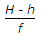# Civil Engineering - Advanced Surveying

### Exercise :: Advanced Surveying - Section 1

16.

If α, H, A and δ be the altitude, hour angle, azimuth and declination of a circumpolar star at its elongation, in latitude λ, the following relation holds good

 A.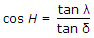B.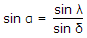C.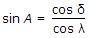D. all the above.

Explanation:

No answer description available for this question. Let us discuss.

17.

Places having same latitude

 A. lie on the parallel of the latitude B. are equidistant from the nearer pole C. are equidistant from both the poles D. are equidistant from the equator E. all the above.

Explanation:

No answer description available for this question. Let us discuss.

18.

The relation between the air base (B), photographic base (b), flying height (H) and the focal length (f) of a vertical photograph, is

 A.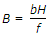B.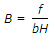C.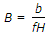D.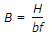Explanation:

No answer description available for this question. Let us discuss.

19.

The difference in longitude of two places expressed in time is equal to the difference in their

 A. sidereal time B. apparent solar time C. mean solar time D. all the above.

Explanation:

No answer description available for this question. Let us discuss.

20.

The scale of a vertical photograph of focal length f taken from height of H metres above M.S.L., at a point of reduced level h, is

 A.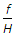B.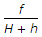C.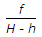D.